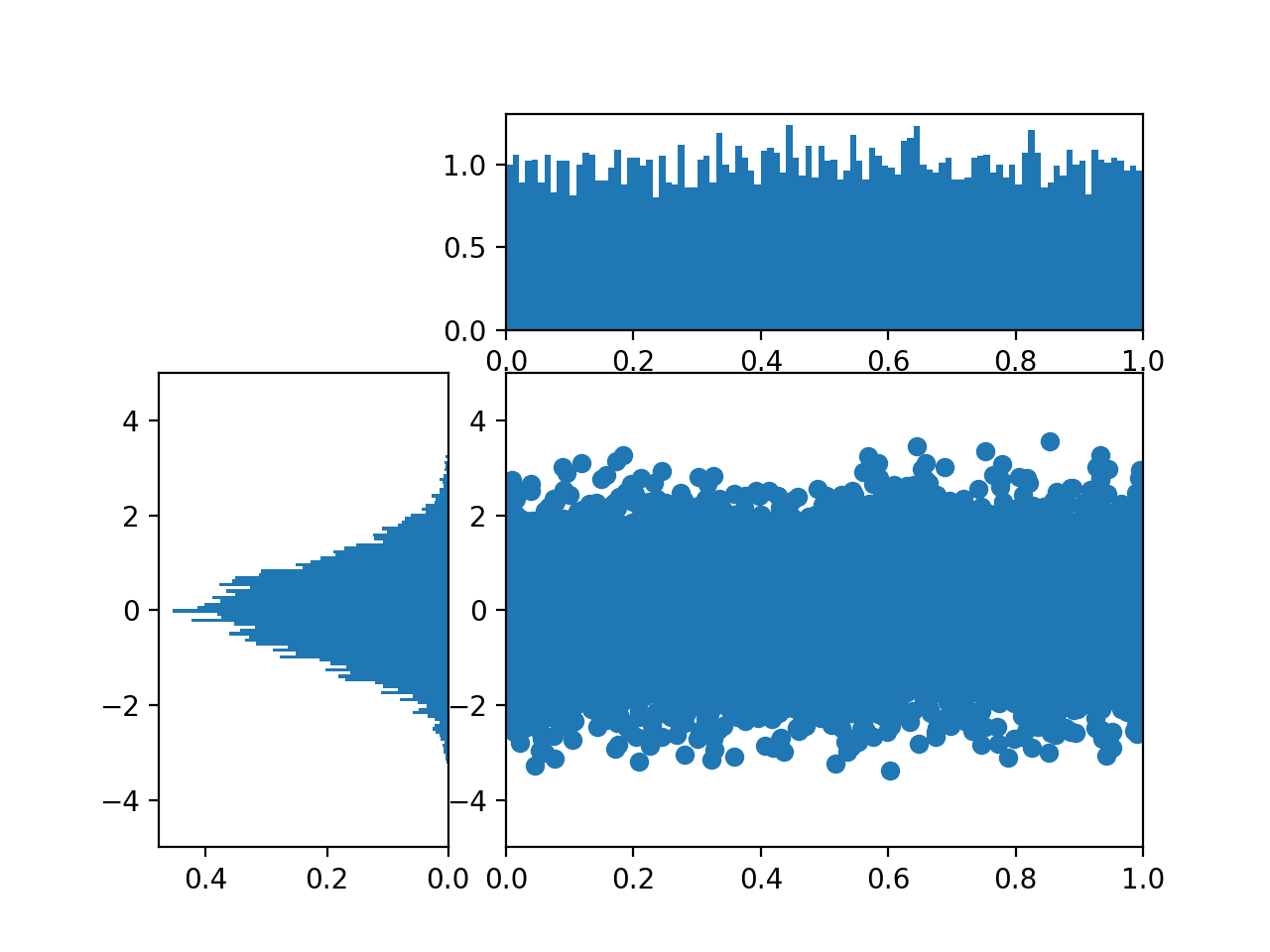# Histograms

A histogram is a type of bar chart that shows the frequency of a phenomena.

An example of a type of histogram are probability distributions. A few examples of probability distributions are random, uniform, normal and chi squared distributions.

These probability functions can be visualized as a curve, where the y-axis holds the probability a given value will occur, and the x-axis is the value itself. This specific type of visual is called a probability density function.

It is possible to plot a given probability distribution by sampling from it, where sampling means that we just pick a number from the distribution at random over and over again. An example is shown below.

%matplotlib notebook

import matplotlib.pyplot as plt
import numpy as np


The np.normal function creates a list of numbers based on the normal distribution. For example:

list(np.random.normal(loc=0.0,
scale=1.0,
size=5).round(3))

[-0.941, -0.95, -0.963, 1.092, 1.213]

fig, ((ax1, ax2), (ax3, ax4)) = plt.subplots(2, 2,
sharex=True)
axs = [ax1,ax2,ax3,ax4]

for n in range(0,len(axs)):
sample_size = 10**(n+1)
sample = np.random.normal(loc=0.0,
scale=1.0,
size=sample_size)
axs[n].hist(sample)
axs[n].set_title('n={}'.format(sample_size))

<IPython.core.display.Javascript object>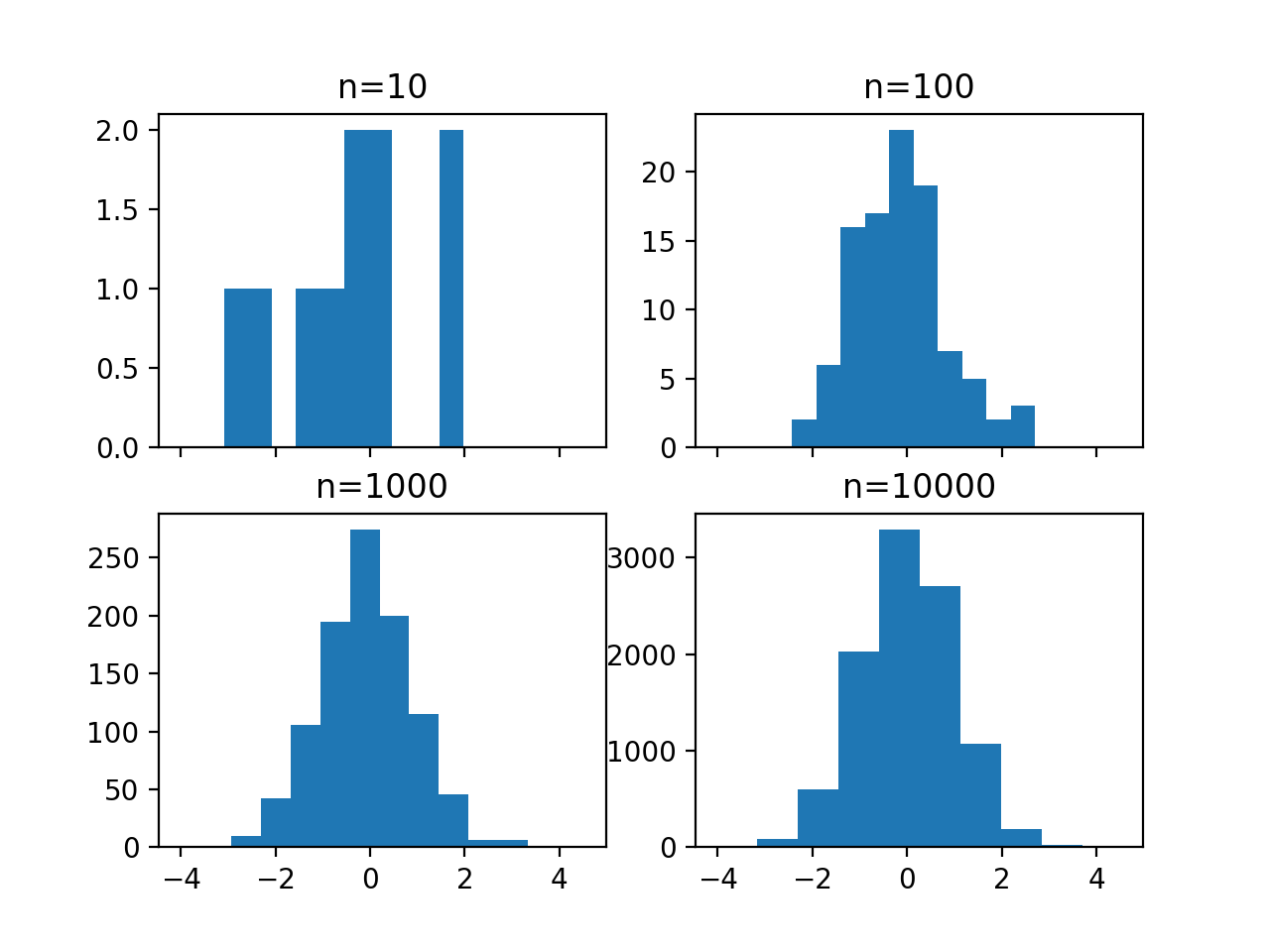By default, matplotlib uses ten bins, which is why the bars appear so skinny for n=10. Ten bins, for n=10, is capturing at best ten unique values. For n=10,000, many values have to be combined into a single bin.

The plots look very different when using 100 bins.

fig, ((ax1, ax2), (ax3, ax4)) = plt.subplots(2, 2, sharex=True)
axs = [ax1,ax2,ax3,ax4]

for n in range(0,len(axs)):
sample_size = 10**(n+1)
sample = np.random.normal(loc=0.0, scale=1.0, size=sample_size)
axs[n].hist(sample, bins=100)
axs[n].set_title('n={}'.format(sample_size))

<IPython.core.display.Javascript object>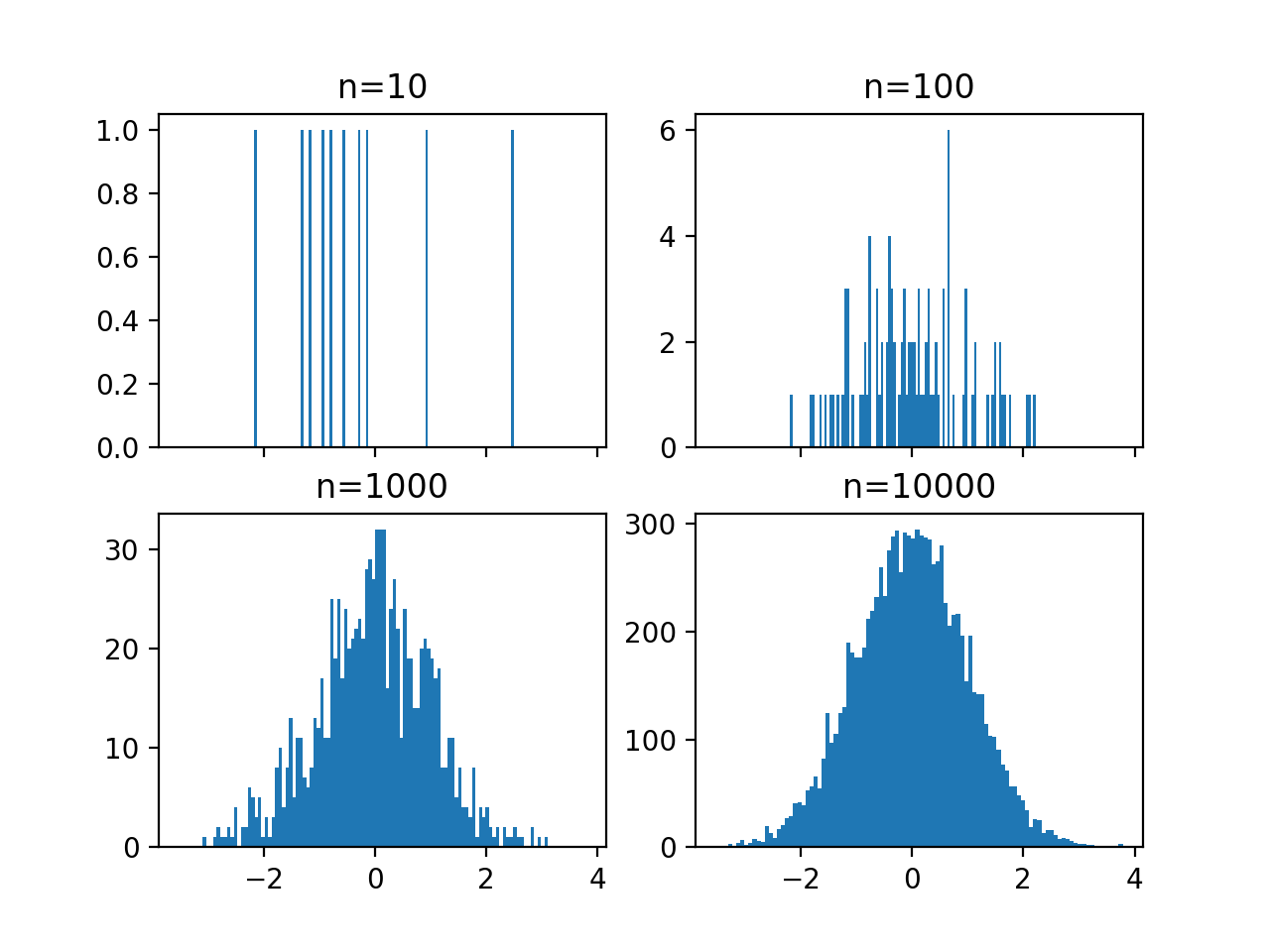#### How many bins should you use in a histogram?

The answer isn’t clear. All of the plots above are “true.” A larger number of bins examines the data at a fine granularity, and a smaller number at a coarse granularity.

This is conceptually similar to using aggregate statistics like the mean and standard deviation to describe a sample of a population. These values are of necessity coarse and whether they are appropriate depends on the users questions and interests.

So, again there’s no real right or wrong number of bins, just useful and useless.

### Gridspec Layout for Subplots

In the following scatterplot, the y-dimension is sampled from a normal distribution and the x-dimension is sampled from a random distribution. This isn’t clear from the scatterplot, but adding two additional plots, both histograms, would make this more clear.

plt.figure()
Y = np.random.normal(loc=0.0, scale=1.0, size=10000)
X = np.random.random(size=10000)
plt.scatter(X,Y);

<IPython.core.display.Javascript object>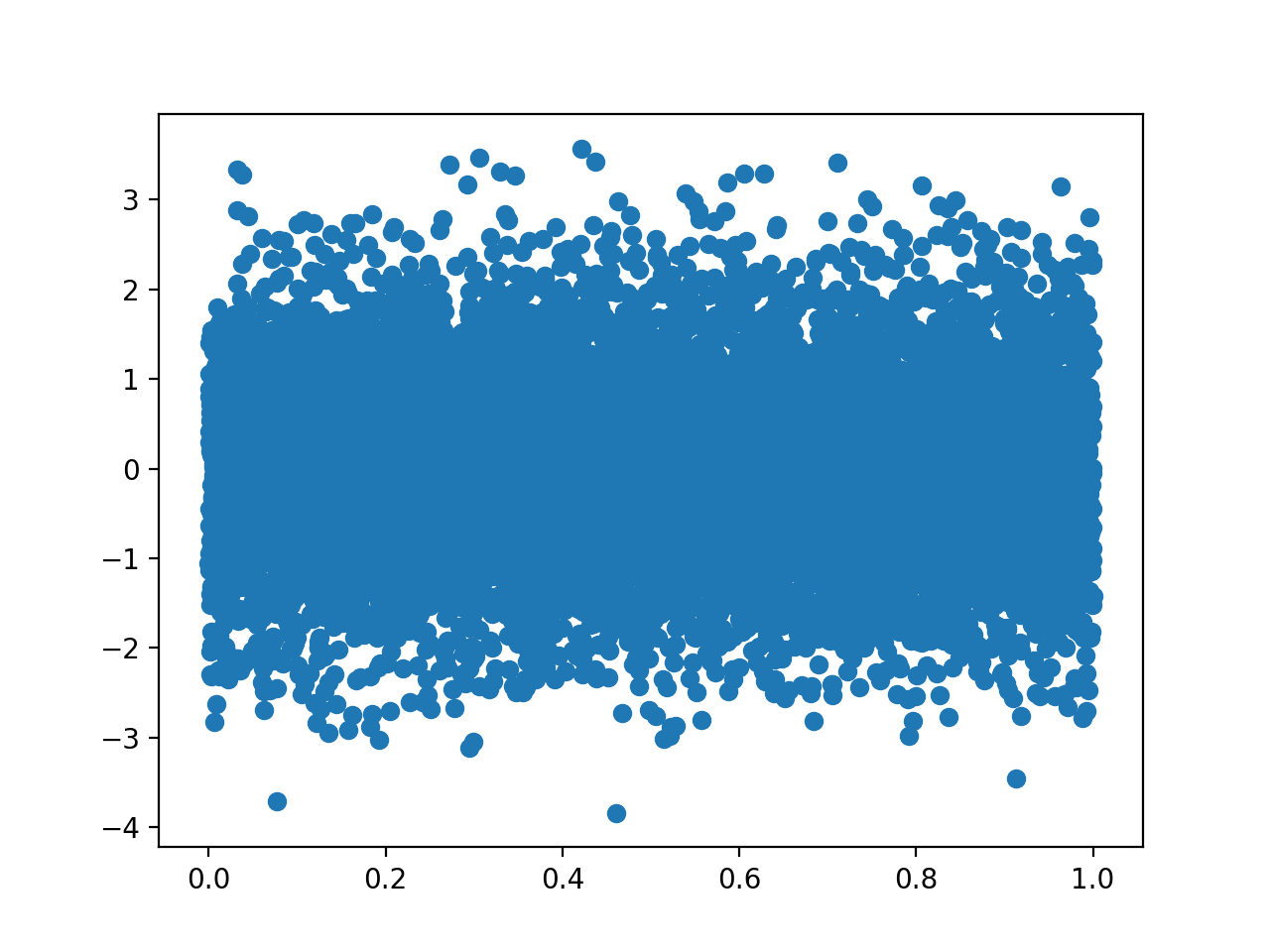In the subplot below:

• The top histogram takes up the top right two grid spaces.
• The histogram on the left side takes up the bottom, left two grid spaces.
• The original scatter plot takes up a two by two square in the bottom right.
import matplotlib.gridspec as gridspec

plt.figure()
gspec = gridspec.GridSpec(3, 3)

top_histogram = plt.subplot(gspec[0, 1:])
side_histogram = plt.subplot(gspec[1:, 0])
lower_right = plt.subplot(gspec[1:, 1:])

<IPython.core.display.Javascript object>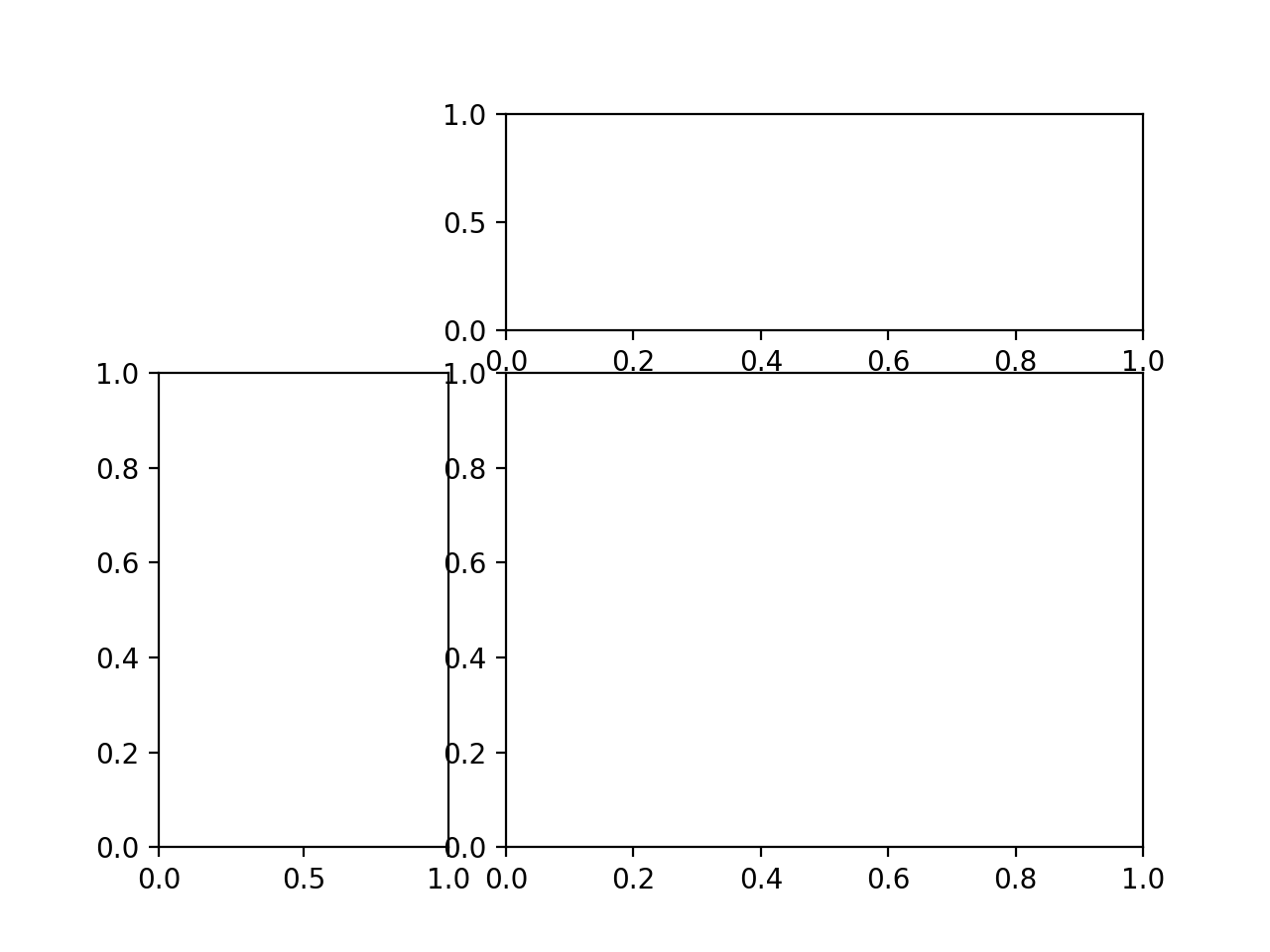Now, fill with data and make some formatting tweaks.

The density parameter tells matplotlib to scale the frequency data in the histogram between 0 and 1. invert_xaxis flips the x-axis, as expected. We cannot make the axes shared at this stage, but we can set them to be the same values, which has the same effect.

plt.figure()
gspec = gridspec.GridSpec(3, 3)

top_histogram = plt.subplot(gspec[0, 1:],
)
side_histogram = plt.subplot(gspec[1:, 0])
lower_right = plt.subplot(gspec[1:, 1:])

Y = np.random.normal(loc=0.0,
scale=1.0,
size=10000)
X = np.random.random(size=10000)
lower_right.scatter(X, Y)
top_histogram.hist(X,
bins=100,
density=True)
s = side_histogram.hist(Y,
bins=100,
orientation='horizontal',
density=True)
side_histogram.invert_xaxis()

for ax in [top_histogram, lower_right]:
ax.set_xlim(0, 1)
for ax in [side_histogram, lower_right]:
ax.set_ylim(-5, 5)

<IPython.core.display.Javascript object>